If there is one prayer that you should pray/sing every day and every hour, it is the LORD's prayer (Our FATHER in Heaven prayer)
It is the most powerful prayer. A pure heart, a clean mind, and a clear conscience is necessary for it.
- Samuel Dominic Chukwuemeka

For in GOD we live, and move, and have our being. - Acts 17:28

The Joy of a Teacher is the Success of his Students. - Samuel Dominic Chukwuemeka

# R and RStudio for Scatter Diagrams## Concepts: Scatter Diagrams; Linear Correlation

(1.) Please begin from Question (1.).
Do not skip.

(2.) Two types of solutions will be given for Question (1.)
The rest of the questions will be done using one type of solution.

(3.) These steps and solutions are for Statistics students.
There are more detailed steps and solutions that I could use for Data Science and Computer Science students.

(1.) The table provided below shows paired data for the heights of a certain​ country's presidents and their main opponents in the election campaign.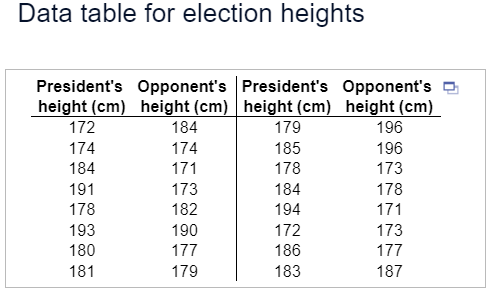(a.) Construct a scatterplot.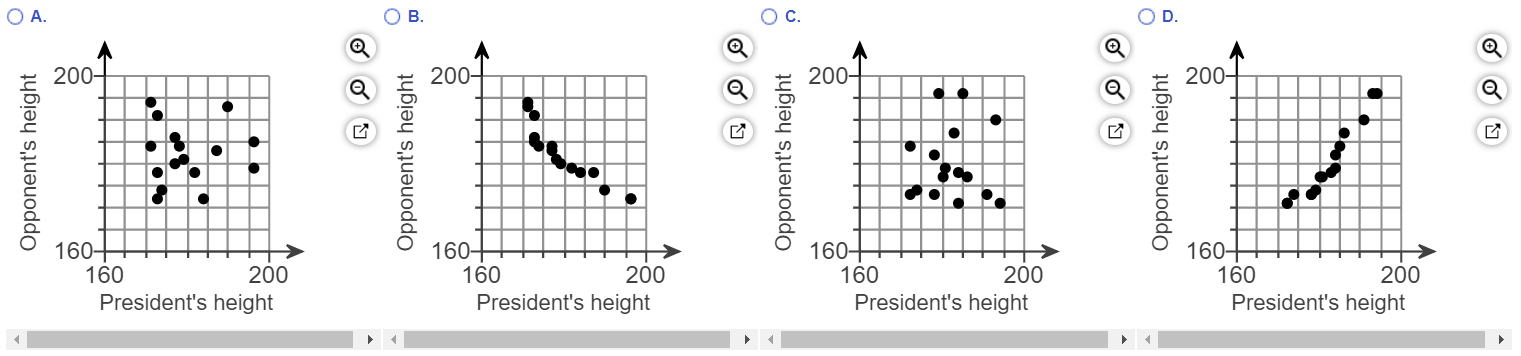(b.) Does there appear to be a correlation between the​ president's height and his​ opponent's height?
A. Yes, there appears to be a correlation. As the​ president's height​ increases, his​ opponent's height decreases.
B. Yes, there appears to be a correlation. As the​ president's height​ increases, his​ opponent's height increases.
C. Yes, there appears to be a correlation. The candidate with the highest height usually wins.
D. No, there does not appear to be a correlation because there is no general pattern to the data.

(1.) Step 1: Open the dataset in Excel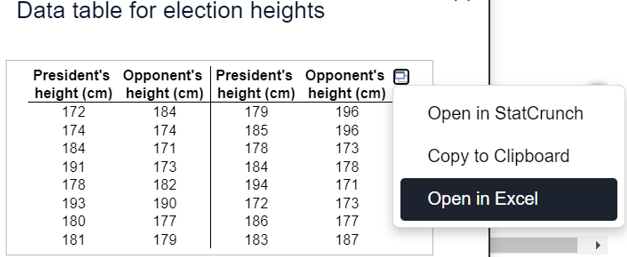(2.) Step 2: Save as a text file
(a.)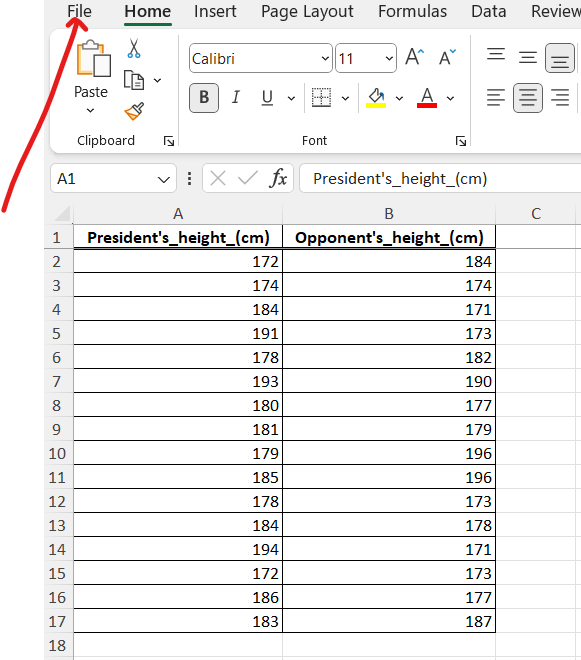(b.)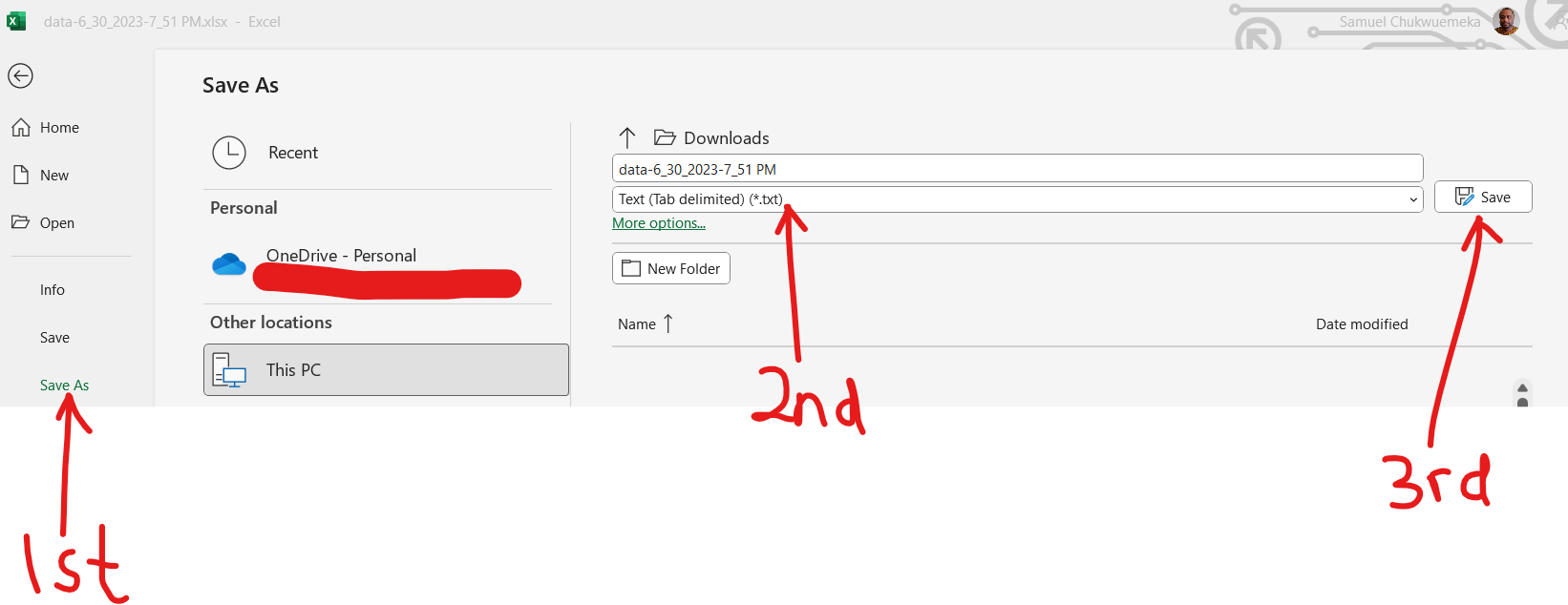(3.) Step 3: Open the text file in RStudio
(a.)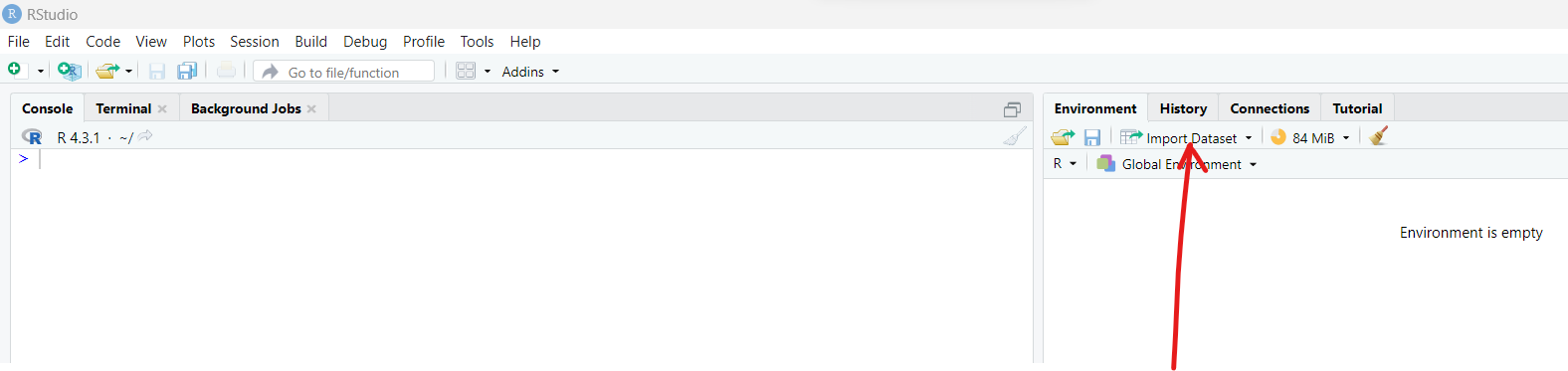(b.)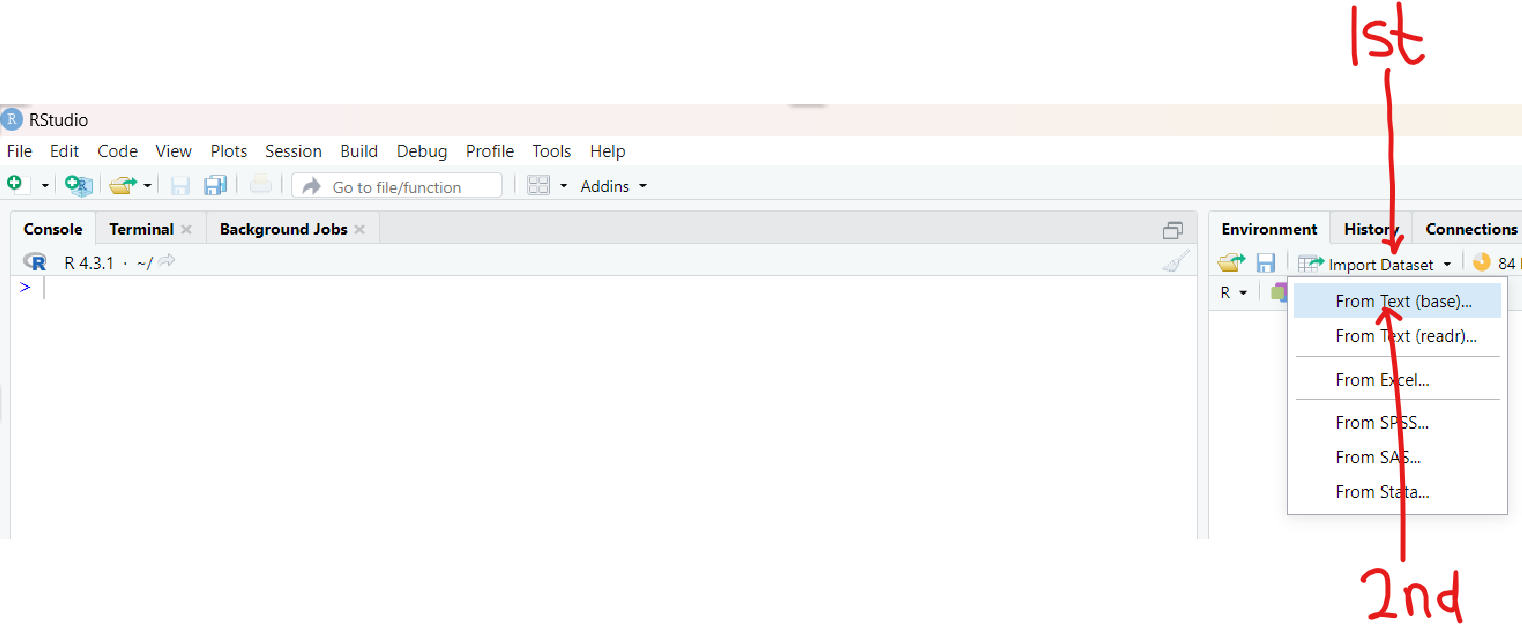(c.)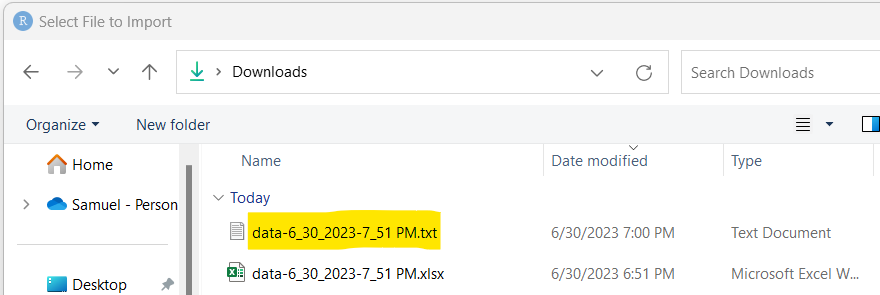(d.)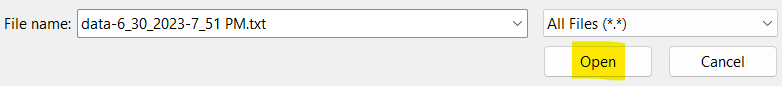(e.)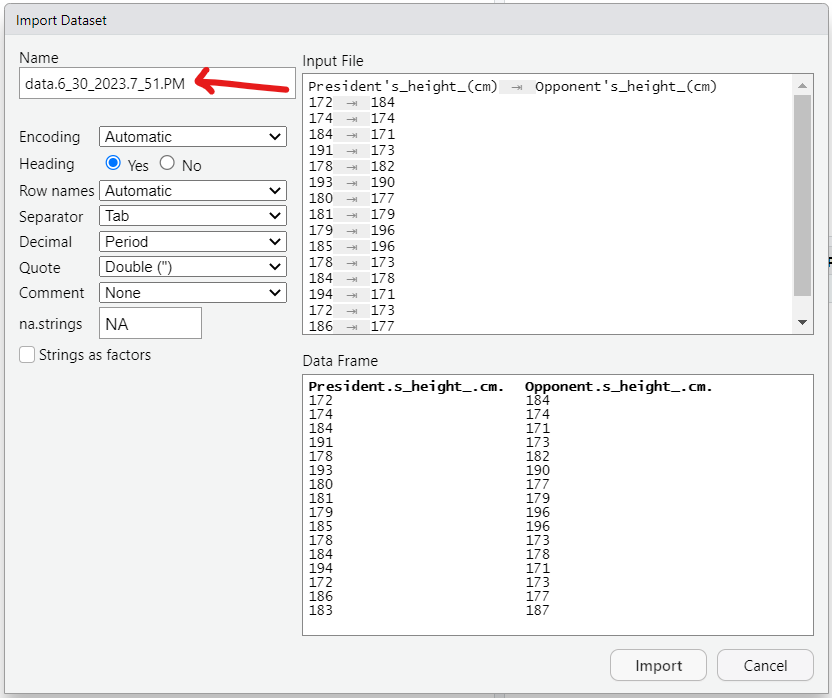(4.) Step 4: Rename the file with a suitable file name and import it into RStudio
(a.)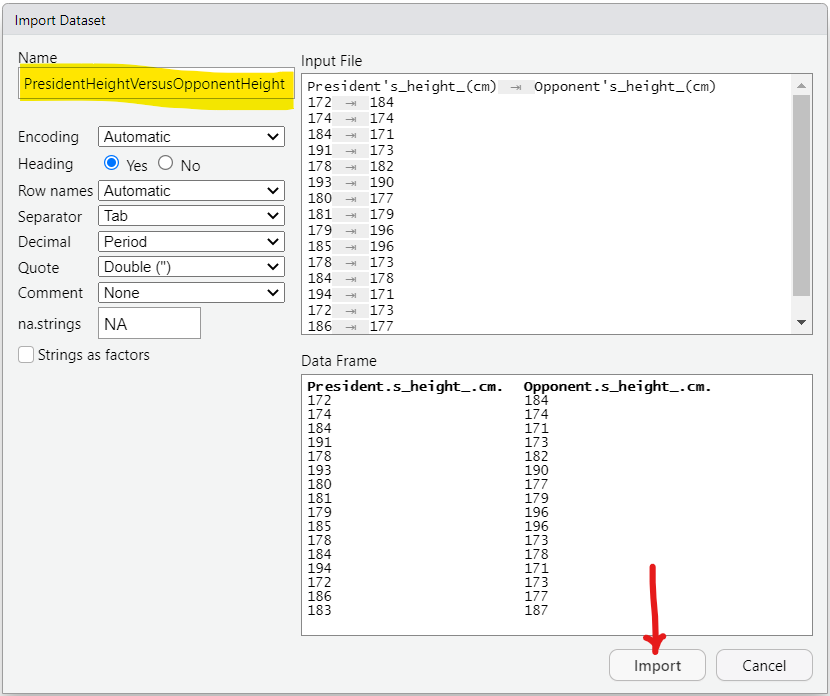I used the file name: PresidentHeightVersusOpponentHeight
This is easier because I can connect it with XaxisVersusYaxis
The x-axis is the President's Height
The y-axis is the Opponent's Height
It is highly recommended to use meaningful file names in the context of the data.
(b.)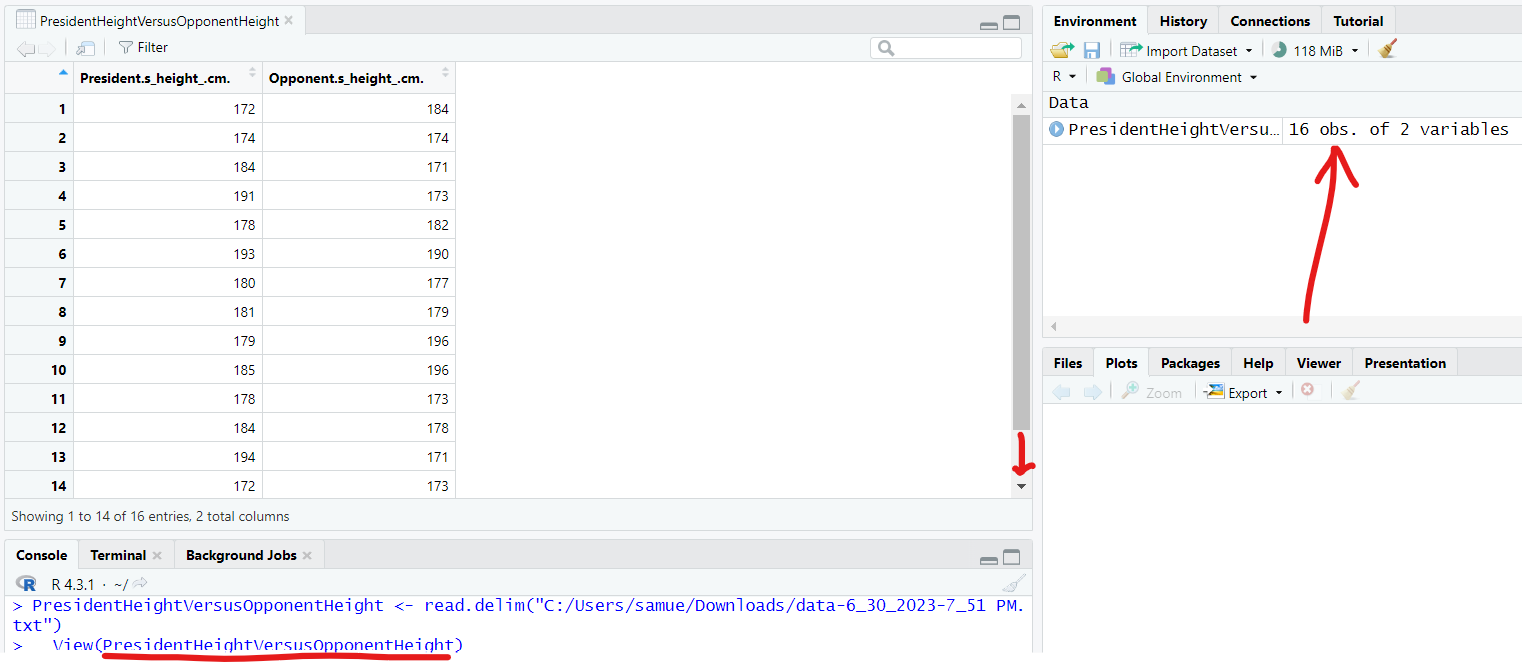As we can see, there are 16 obs (observations) and 2 variables in the PresidentHeightVersusOpponentHeight dataset.

(5.) 1st Solution: plot function with only one argument
The function is plot
The argument is the file name: PresidentHeightVersusOpponentHeight
By default, RStudio displays first variable (variable in the first column) as the x-axis and the second variaable (variable in the second column) as the y-axis.
This is a quick and easy solution
In the console window, type the command:
                        plot(PresidentHeightVersusOpponentHeight)

(a.)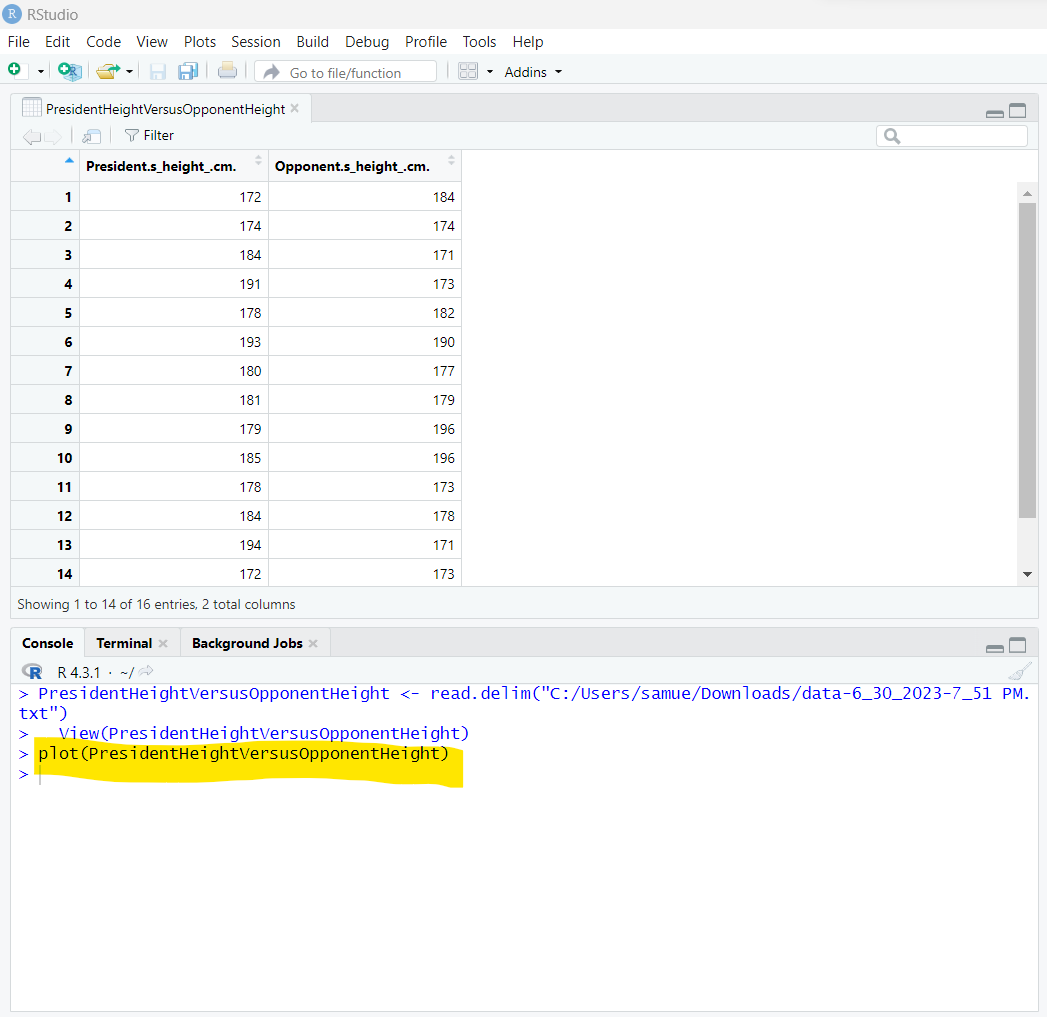(b.)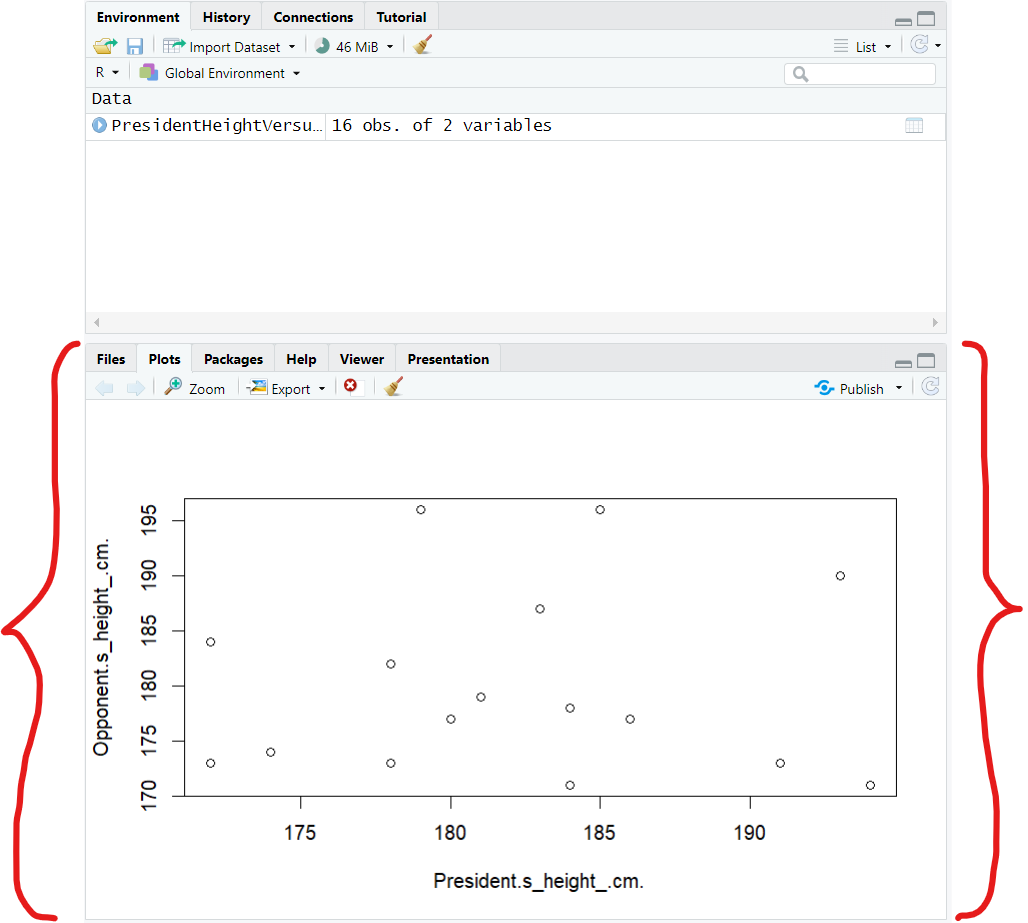But here's the reason why we need more arguments:
(I.) Some people may be confused whether the correct option is Option A. or Option C.
Although after expanding both options and carefully comparing them with the RStudio graph, you may see the correct option.
Be it as it may, we want the graph in RStudio to exactly match the correct one in the option.
The minimum and maximum values used on the graphs in the options are different from the minimum and maximum values on the graph in RStudio
So, it is better we use adjust the one in RStudio to match the one in the options.
We shall use the arguments, each separated by a comma:
                        xlim = c(160, 200)
ylim = c(160, 200)

where:
xlim is the limit for the x-axis. This includes the minimum value and the maximum value for the x-axis
ylim is the limit for the y-axis. This includes the minimum value and the maximum value for the y-axis
c is the function that selects and combines the values into a list. It is used when we need to pass a list (in this case: the values in both axis) as a parameter.

(II.) The points on the graph in RStudio are circles (open cirles) while the ones in the options are filled circles (closed circles).
By default, RStudio displays the points as open circles. But we want filled/shaded circles.
To fix this, we shall use the argument:
pch = 16
where:
pch is the Plot Character
pch = 16 is the value of the plot character for filled circle

(III.) The labels on the graph in the options are not exactly the same from the those in the RStudio graph
To label the one in RStudio accordingly, we use the argument:
                        xlab = "President's height"
ylab = "Opponent's height"

(6.) 2nd Solution: Let us use more arguments (the ones we just listed) with the plot function

 plot(PresidentHeightVersusOpponentHeight, xlab = "President's height", ylab = "Opponent's height", xlim = c(160, 200), ylim = c(160, 200), pch = 16) 

(a.)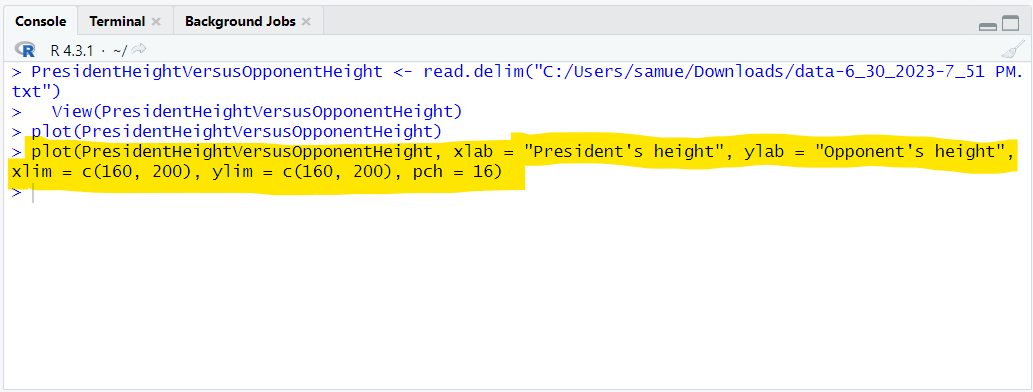(b.)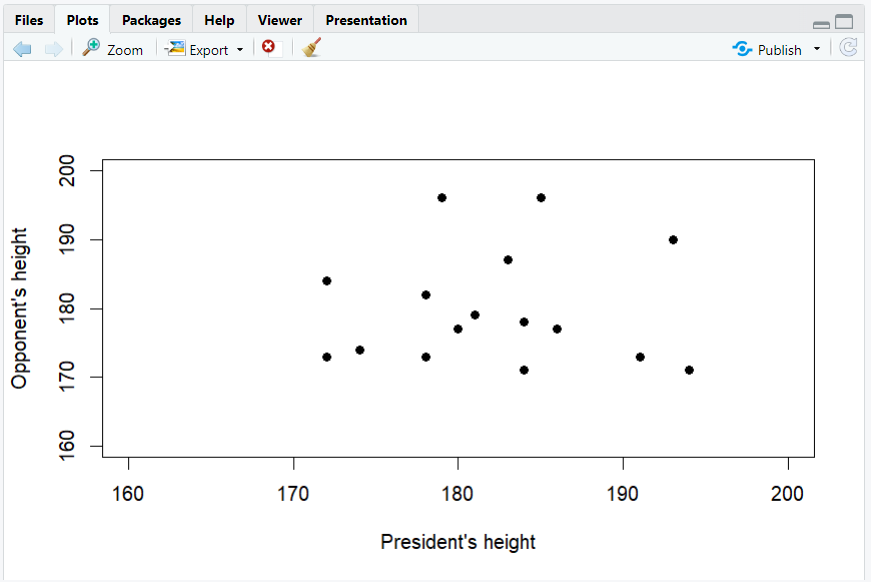We now see that the correct option is Option C.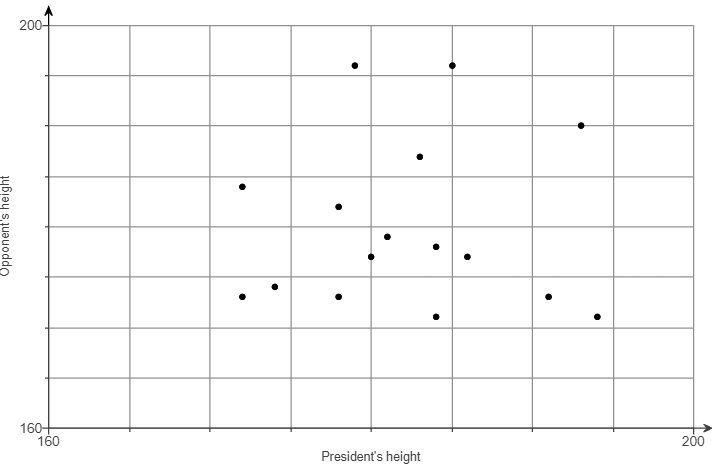The points are scattered. There is no clear trend.
Hence, there does not appear to be a correlation because there is no general pattern to the data.

(2.) The table lists weights​ (pounds) and highway mileage amounts​ (mpg) for seven automobiles.

 Weight (lb) 3185 3420 3835 4465 4650 2140 3745 Highway (mpg) 32 30 26 22 21 39 28

(a.) Use the sample data to construct a scatterplot.
Use the first variable for the​ x-axis.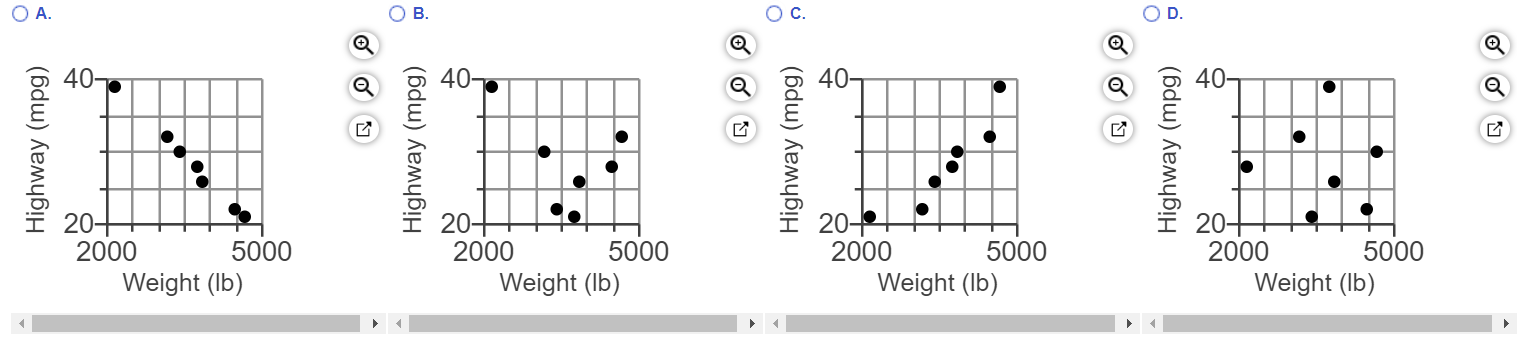(b.) Is there a linear relationship between weight and highway​ mileage?
A. No, there appears to be no relationship.
B. No, there appears to be a​ relationship, but it is not linear.
C. ​Yes, as the weight increases the highway mileage decreases.
D. Yes, as the weight increases the highway mileage increases.

(a.) The code to draw the scatter diagram to match exactly one of the options is:

 plot(WeightVersusHighway, xlab = "Weight (lb)", ylab = "Highway (mpg)", xlim = c(2000, 5000), ylim = c(20, 40), pch = 16) 

(I.)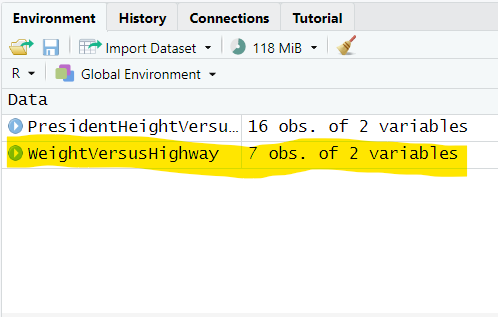(II.)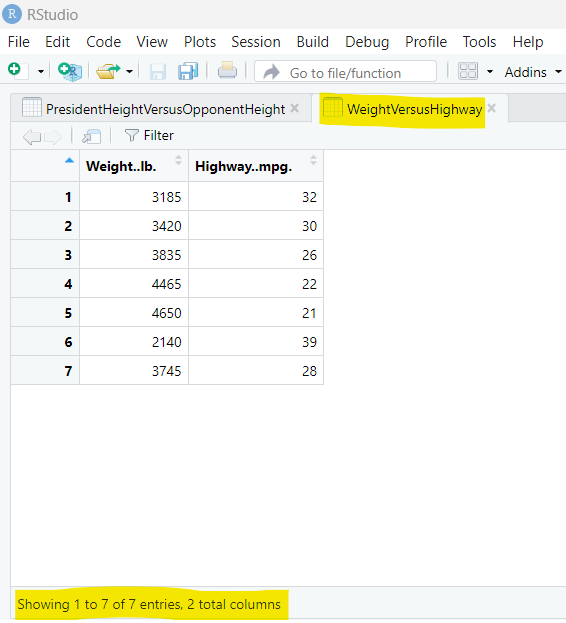(III.)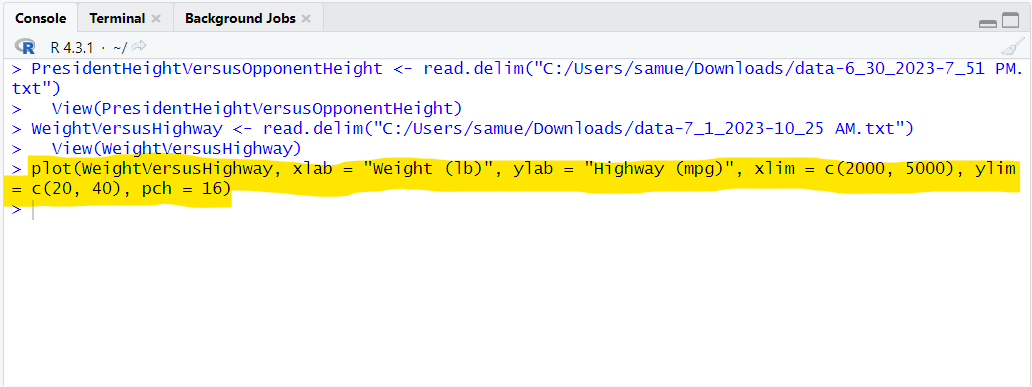(IV.)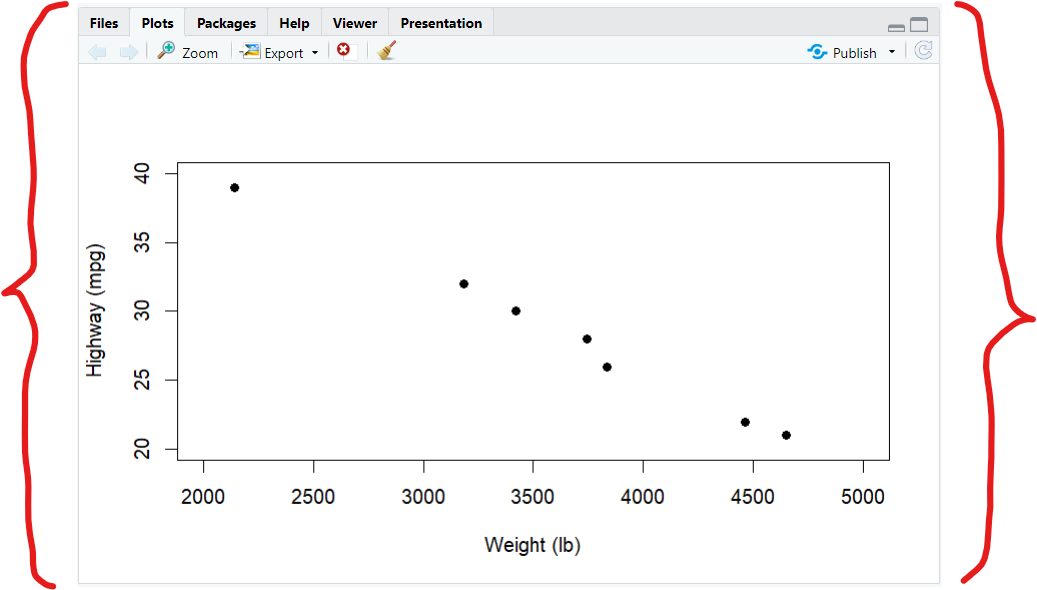We see that the correct option is Option A.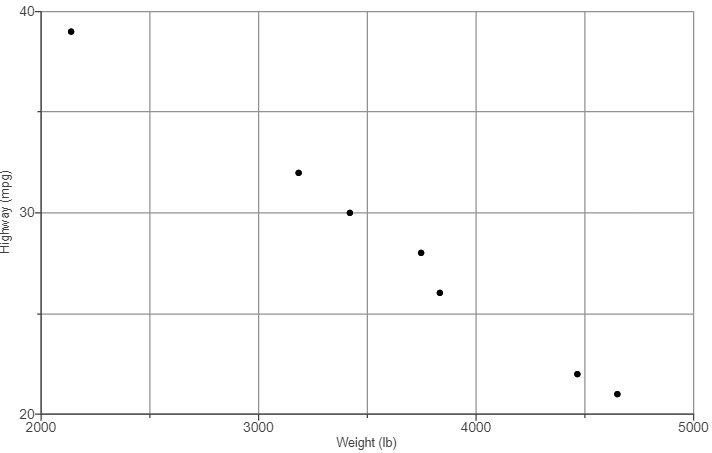(b.) There is a pattern in the scatterplot.
It is a negative trend.
It shows a negative slope.
This implies that as the weight increases the highway mileage decreases.

(3.) This data is from a study comparing the amount of tar and carbon monoxide​ (CO) in cigarettes.
Use tar for the horizontal scale and use carbon monoxide​ (CO) for the vertical scale.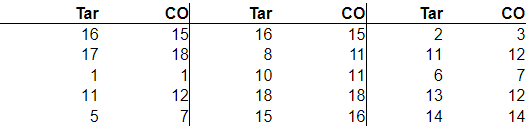(a.) Construct a scatterplot.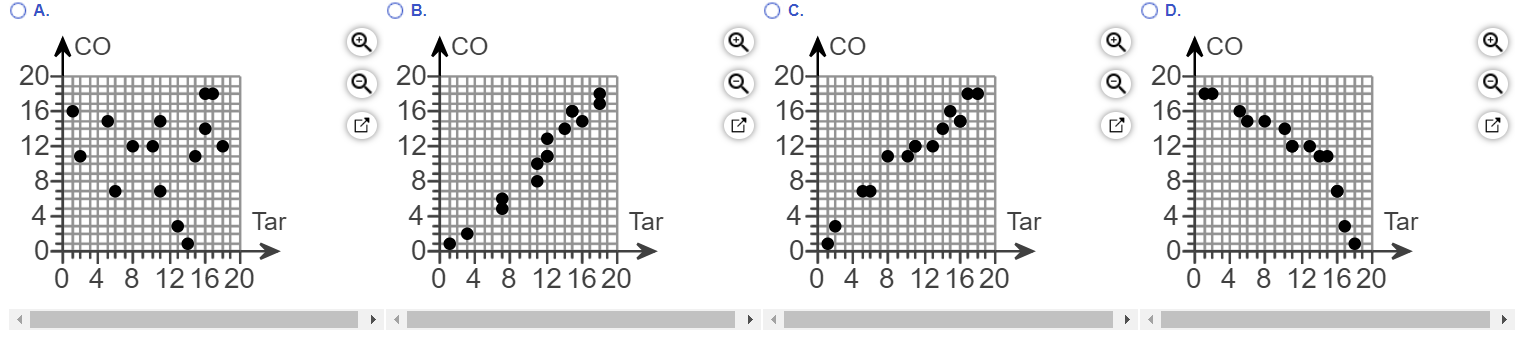(b.) Is there a relationship between cigarette tar and​ CO?
A. ​Yes, as the amount of tar increases the amount of carbon monoxide decreases.
B. Yes, as the amount of tar increases the amount of carbon monoxide also increases.
C. ​No, there appears to be no relationship.

(a.) The code to draw the scatter diagram to match one of the options is:

 plot(TarVersusCO, xlab = "Tar", ylab = "CO", xlim = c(0, 20), ylim = c(0, 20), pch = 15) 

(I.)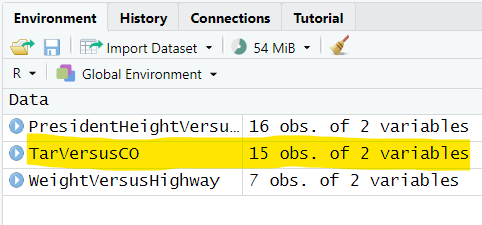(II.)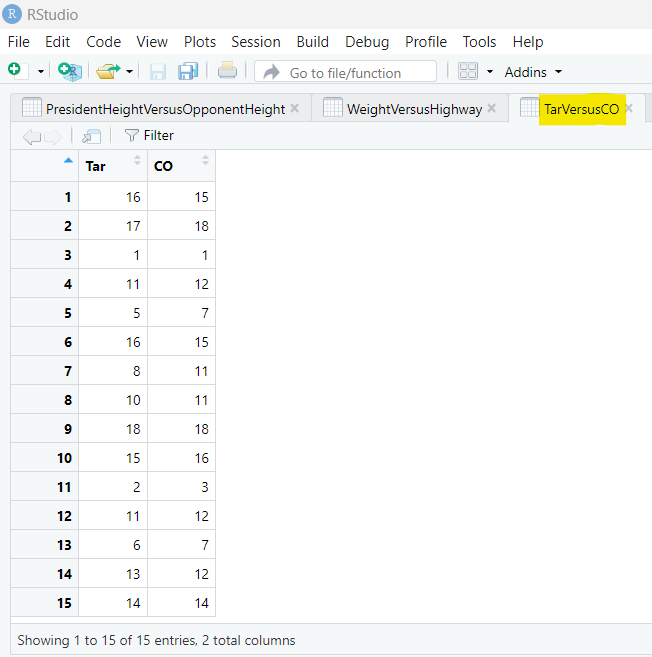(III.)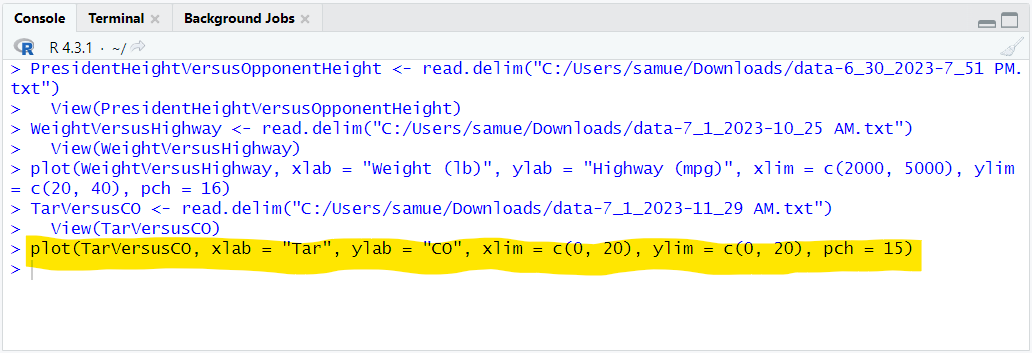(IV.)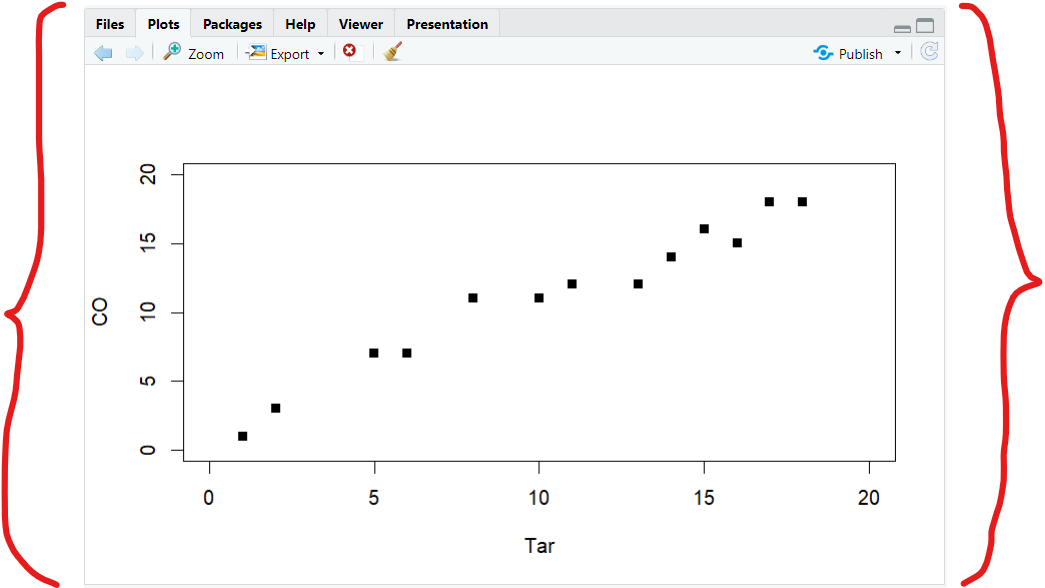We see that the correct option is Option C.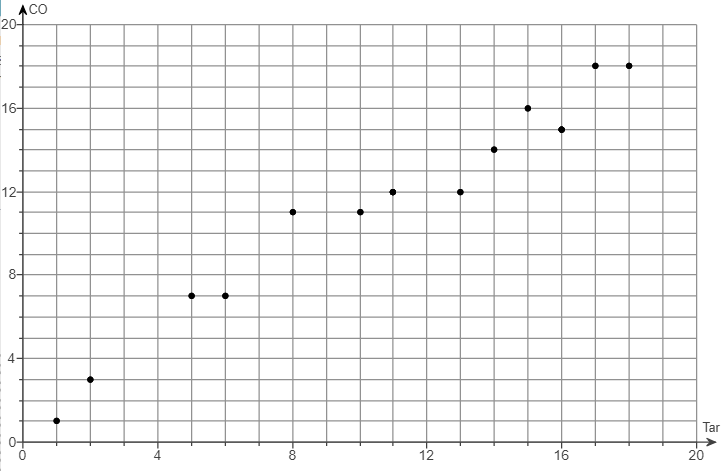(b.) There is a pattern in the scatterplot.
It is a positive trend.
It shows a positive slope.
This implies that as the amount of tar increases the amount of carbon monoxide also increases.

Plot Character is the argument that sets the characters/points in a plot.
It is denoted by pch
It has numeric values that shows several symbols used to represent the points in a plot.

Plot Characters for RStudio
Value Symbol Argument
0 Square pch = 0
1 Circle pch = 1
2 Triangle: Vertex up pch = 2
3 Plus pch = 3
4 Cross pch = 4
5 Diamond pch = 5
6 Triangle: Vertex down pch = 6
7 Cross inside Square (Square Cross) pch = 7
8 Asterisk pch = 8
9 Plus inside Diamond (Diamond Plus) pch = 9
10 Plus inside Circle (Circle Plus) pch = 10
11 Two Triangles: Vertex up and down pch = 11
12 Plus inside Square (Square Plus) pch = 12
13 Cross inside Circle (Circle Cross) pch = 13
14 Triangle: Vertex up inside Square pch = 14
15 Filled/Shaded Square pch = 15
16 Filled/Shaded Circle pch = 16
17 Filled/Shaded Triangle: Vertex up pch = 17
18 Filled/Shaded Diamond pch = 18
19 Filled/Shaded Circle pch = 19
20 Small Shaded Circle pch = 20
21 Circle pch = 21
22 Square pch = 22
23 Diamond pch = 23
24 Triangle: Vertex up pch = 24
25 Triangle: Vertex down pch = 25